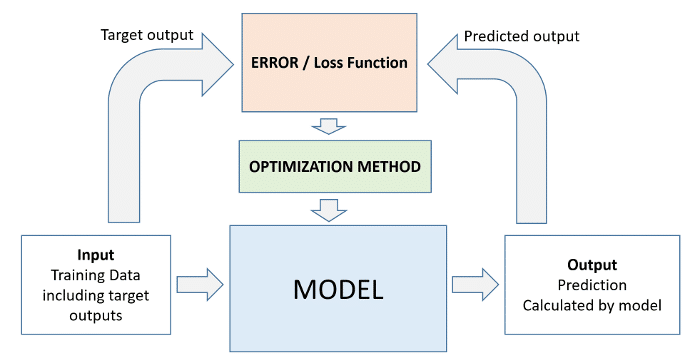# .css-4zleql{display:block;}ProgrammingFire# Which Machine Learning algorithm to use in different ML problems?

Nouman Rahman
·Aug 19, 2022·

Subscribe to my newsletter and never miss my upcoming articles

• Introduction
• Logistic Regression vs. Linear Regression
• Identify Classification or Regression problems
• Linear Regression for Solving Regression Problems
• Logistic Regression for Solving Classification Problems
• ML Workflow
• Next Steps

## Introduction

Logistic Regression and Linear Regression are two different Machine Learning algorithms used for different Machine Learning problems. So, First, we need to know about ML.

Machine learning is simply the process of computing the best parameters to model the relationship between some feature and targets.

Every machine learning problem has three components:

• Model
• Cost Function
• Optimizer

## Logistic Regression vs. Linear Regression

Linear Regression: It is used for regression problems Logistic Regression: It is used for classification problems

## Identify Classification or Regression problems

Identifying whether a given problem is a classification or regression problem is an important first step in machine learning.

### Classification Problems

Problems where each input must be assigned a discrete category (also called label or class) are known as classification problems.

Here are some examples of classification problems:

• Rainfall prediction: Predicting whether it will rain tomorrow using today's weather data (classes are "Will Rain" and "Will Not Rain")
• Breast cancer detection: Predicting whether a tumor is "benign" (noncancerous) or "malignant" (cancerous) using information like its radius, texture, etc.
• Loan Repayment Prediction - Predicting whether applicants will repay a home loan based on factors like age, income, loan amount, no. of children, etc.
• Handwritten Digit Recognition - Identifying which digit from 0 to 9 a picture of handwritten text represents.

### Regression Problems

Problems, where a continuous numeric value must be predicted for each input, are known as regression problems.

Here are some examples of regression problems:

• Medical Charges Prediction
• House Price Prediction
• Ocean Temperature Prediction
• Weather Temperature Prediction

## Linear Regression for Solving Regression Problems

Linear regression is a commonly used technique for solving regression problems. In a linear regression model, the target is modeled as a linear combination (or weighted sum) of input features. The predictions from the model are evaluated using a loss function like the Root Mean Squared Error (RMSE).

Here's a visual summary of how a linear regression model is structured:## Logistic Regression for Solving Classification Problems

Logistic regression is a commonly used technique for solving binary classification problems. In a logistic regression model:

• we take linear combination (or weighted sum of the input features)
• we apply the sigmoid function to the result to obtain a number between 0 and 1
• this number represents the probability of the input being classified as "Yes"
• instead of RMSE, the cross entropy loss function is used to evaluate the results

Here's a visual summary of how a logistic regression model is structured:The sigmoid function applied to the linear combination of inputs has the following formula:The output of the sigmoid function is called a logistic, hence the name logistic regression. Logistic regression can also be applied to multi-class classification problems, with a few modifications.

## ML Workflow

Whether we're solving a regression problem using linear regression or a classification problem using logistic regression, the workflow for training a model is exactly the same:

• We initialize a model with random parameters (weights & biases).
• We pass some inputs into the model to obtain predictions.
• We compare the model's predictions with the actual targets using the loss function.
• We use an optimization technique (like least squares, gradient descent, etc.) to reduce the loss by adjusting the weights & biases of the model
• We repeat steps 1 to 4 till the predictions from the model are good enough.Classification and regression are both supervised machine learning problems because they use labeled data. Machine learning applied to unlabeled data is known as unsupervised learning.## Next Steps

• Train a Linear Regression model using one of these datasets:

• Train a Logistic Regression model using one of these datasets:

### Did you find this article valuable?

Support Nouman Rahman by becoming a sponsor. Any amount is appreciated!